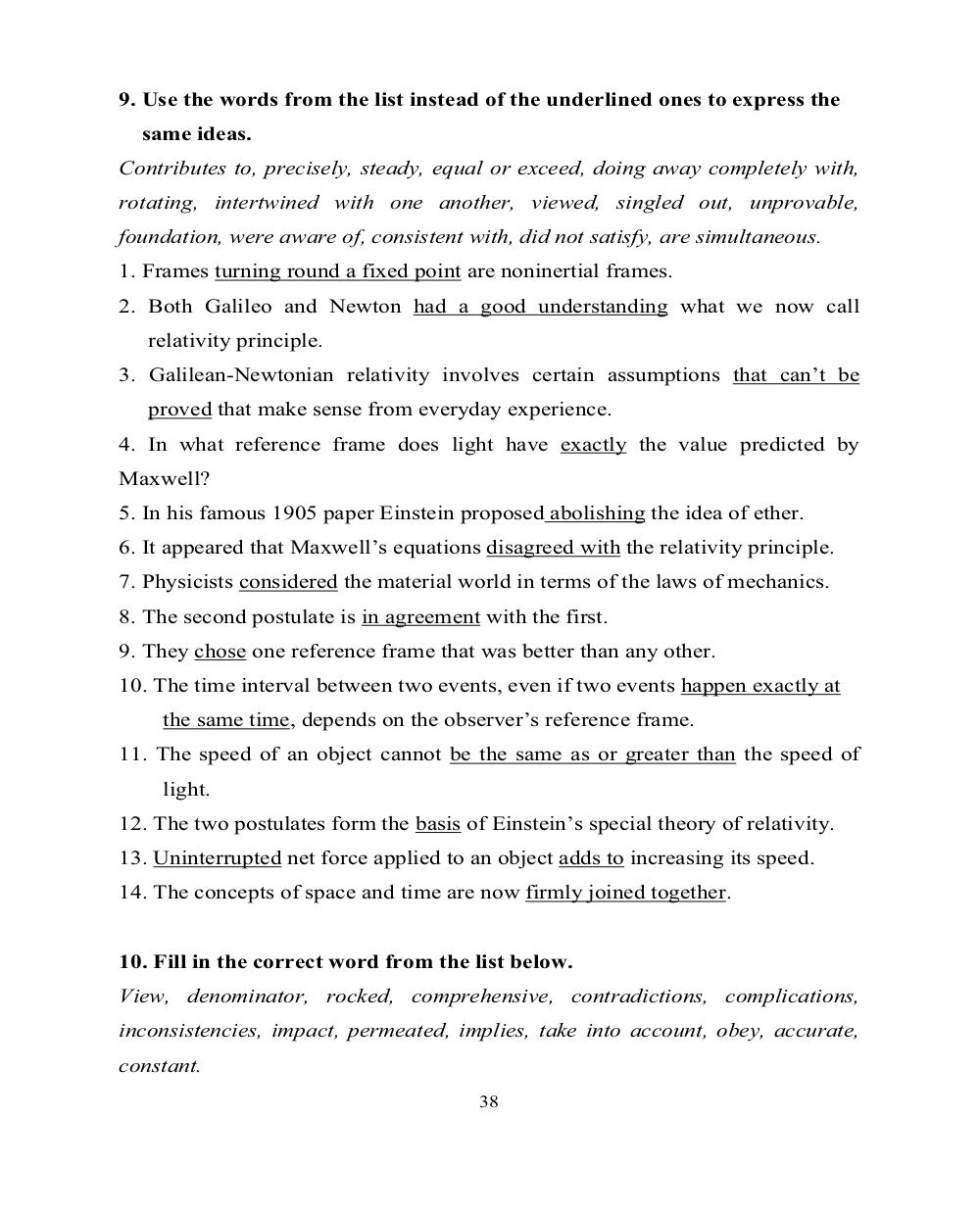# Английский язык. Ч.3 (Units 9,10). Ильичева Н.А - 38 стр.

Составители:

Рубрика:

• ## Иностранный язык9. Use the words from the list instead of the underlined ones to express the
same ideas.
Contributes to, precisely, steady, equal or exceed, doing away completely with,
rotating, intertwined with one another, viewed, singled out, unprovable,
foundation, were aware of, consistent with, did not satisfy, are simultaneous.
1. Frames
turning round a fixed point
are noninertial frames.
2. Both Galileo and Newton had a good understanding
what we now call
relativity principle.
3. Galilean-Newtonian relativity involves certain assumptions that can’t be
proved that make sense from everyday experience.
4. In what reference frame does light have exactly
the value predicted by
Maxwell?
5. In his famous 1905 paper Einstein proposed abolishing
the idea of ether.
6. It appeared that Maxwell’s equations disagreed with
the relativity principle.
7. Physicists considered
the material world in terms of the laws of mechanics.
8. The second postulate is in agreement
with the first.
9. They chose
one reference frame that was better than any other.
10. The time interval between two events, even if two events happen exactly at
the same time, depends on the observer’s reference frame.
11. The speed of an object cannot be the same as or greater than
the speed of
light.
12. The two postulates form the basis
of Einstein’s special theory of relativity.
13. Uninterrupted
net force applied to an object adds to increasing its speed.
14. The concepts of space and time are now firmly joined together
.
10. Fill in the correct word from the list below.
View, denominator, rocked, comprehensive, contradictions, complications,
inconsistencies, impact, permeated, implies, take into account, obey, accurate,
constant.
389. Use the words from the list instead of the underlined ones to express the
same ideas.
Contributes to, precisely, steady, equal or exceed, doing away completely with,
rotating, intertwined with one another, viewed, singled out, unprovable,
foundation, were aware of, consistent with, did not satisfy, are simultaneous.
1. Frames turning round a fixed point are noninertial frames.
2. Both Galileo and Newton had a good understanding what we now call
relativity principle.
3. Galilean-Newtonian relativity involves certain assumptions that can’t be
proved that make sense from everyday experience.
4. In what reference frame does light have exactly the value predicted by
Maxwell?
5. In his famous 1905 paper Einstein proposed abolishing the idea of ether.
6. It appeared that Maxwell’s equations disagreed with the relativity principle.
7. Physicists considered the material world in terms of the laws of mechanics.
8. The second postulate is in agreement with the first.
9. They chose one reference frame that was better than any other.
10. The time interval between two events, even if two events happen exactly at
the same time, depends on the observer’s reference frame.
11. The speed of an object cannot be the same as or greater than the speed of
light.
12. The two postulates form the basis of Einstein’s special theory of relativity.
13. Uninterrupted net force applied to an object adds to increasing its speed.
14. The concepts of space and time are now firmly joined together.

10. Fill in the correct word from the list below.
View, denominator, rocked, comprehensive, contradictions, complications,
inconsistencies, impact, permeated, implies, take into account, obey, accurate,
constant.
38